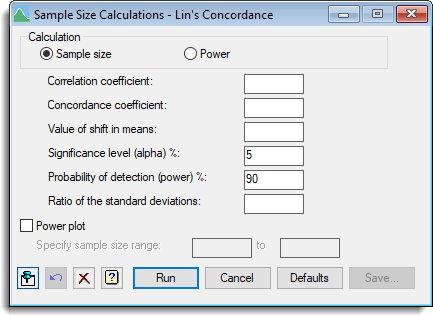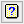1. Home
2. Sample Size Calculations – Lin’s Concordance Coefficient

# Sample Size Calculations – Lin’s Concordance Coefficient

Select menu: Stats | Sample Size | Lin’s Concordance Coefficient

Use this to calculate the number of replicates (sample size) or the power required for Lin’s Concordance Coefficient. Lin’s concordance correlation coefficient can be used to assess how well a new method of measurement reproduces the results provided by a standard method. To do this, you measure the same set of units using the two methods, and calculate the concordance between the resulting two sets of measurements. The methods are regarded as equivalent if the coefficient is greater than some threshold.

Stats | Sample Size | Lin’s Concordance.
2. Fill in the fields as required then click Run.

After running the calculation you can save the results by clicking Save.## Calculation

Specifies the calculation to be carried out: Sample size, or Power.

## Correlation coefficient

Specifies the correlation for two samples with the smallest amount of non-reproducibility required to be detected.

## Concordance coefficient

Specifies the value of Lin’s concordance for two samples with the smallest amount of non-reproducibility required to be detected.

## Value of shift in means

Specifies the value of the shift in means (divided by the harmonic mean of the standard deviations) for two samples with the smallest amount of non-reproducibility required to be detected.

## Significance level (alpha)%

Specifies the significance level at which the response is to be tested.

## Probability of detection (power)%

For a sample size calculation this specifies the required probability for detection of the response (that is, the power of the test).

## Sample size

For a power calculation this specifies the sample size to be used to calculate the probability of detection (power).

## Ratio of the standard deviations

Specifies the value of the ratio of the standard deviations for two samples with the smallest amount of non-reproducibility required to be detected.

## Power plot

When selected, produces a plot of power versus sample size. When Sample size is selected the plot is generated using 11 replication values centred around the required number of replicates for the requested power. When Power is selected you can specify a range of sample size values for the plot in the spaces provided.

## Action IconsPin Controls whether to keep the dialog open when you click Run. When the pin is downthe dialog will remain open, otherwise when the pin is upthe dialog will close.Restore Restore names into edit fields and default settings.Clear Clear all fields and list boxes.Help Open the Help topic for this dialog.